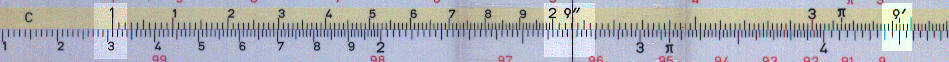Trigonometric calculations (sine and tangent)

Gauge points for small angles

Introduction

On many European rules, though not as many from the USA, you will find gauge points marked on the rule to calculate the sine or tangent of small angles measured in minutes or seconds. For small angles the sine and the tangent are equal.The example, from an Aristo Studio 0968, shows the calculation of the sine of 27 seconds.

Align the r" symbol with the angle (27") and the read answer (0.000131) on the D scale against the 1 on the C scale.

Many rules also have a gauge point, highlighted here in green, for similar calculations with angles in minutes.

The true value of the seconds symbol, r", is 206264, that is 360° x 60' x 60" / (2p). The value of the minutes symbol r' is 3437.7, that is  360° x 60' / (2p). On some French and other European rules you may see another "seconds" symbol at 6366.2, that is 400grad x 100 / (2p).